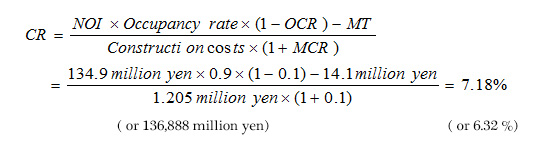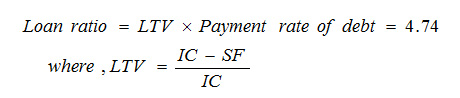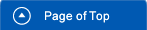Home > Various Aspects > Economic Aspects## Economic Aspects

### 1. General

The total construction costs of the soft-landed water city consist of ①Purchase expense for land (about 40,000 ㎡) of development, ②Destruction and removal expense for existing buildings, ③Soil excavation cost, ④Construction cost for dikes, ⑤Construction cost for floaters with habitation infrastructures, ⑥Building construction cost on floaters, ⑦Common supporting expense, ⑧management expenses and so on. Of these, soil excavation cost will not be prohibitive since it is cheaper to excavate soil in low-lying lands at sea level because the amount of soil to be removed is small. Meanwhile, expensive seawalls are not necessary for construction of the floating foundations in the artificial water area (reservoir) as the influences of waves and currents like open sea need not be taken into account.

In general, the main body for redevelopment is the local government preparing with the purchase expense for land area, the construction expense for reservoirs and so on while the construction cost for floating units should be paid mainly by users. Therefore, the study on the construction cost of floating units become important to carry out. We have carried out an economic study for the construction of a soft-landed water city "WATERPOLIS" - concentrating especially on the cost performance of a floating modular unit. In estimating the construction cost of floating units, their economic efficiency was examined on the basis of the standard cash flow approach.

### 2. Estimation of the initial cost of floating unit

The estimation of construction costs for a floating foundation built of steel has been made using data from the Mega-float project, and it resulted as 276,255 to 363,446 yen/t (figures as of 2000) or about 2,763 to 3,634 U.S. dollars assuming the exchange ratio of 100 yen/U.S. dollar. Based upon such numbers, a single floating modular unit (100 m x 25 m x 4.5 m) for our research purpose will mean a single unit having a construction cost of some 5.194 U.S. million dollars (or 25.97 million U.S. dollars for the 5 modular units). As for the construction cost of housing, etc., according to a case-study information for 12 to 14 low-rise houses (total floor space: 1,120 - 1,440 ㎡) the cost will be 1.784 million U.S. dollars and for high-rise apartments (total floor space: 1,920 ㎡) for 30 families, the cost will be 3.072 million U.S. dollars (figures as of 2005). Thus, for those buildings atop the floating unit, the cost in total will be 4.856 million U.S. dollars. In addition, the cost for utility facilities and/or fixtures such as water pipes, pumps and electric cables can be estimated to be 2.00 million U.S. dollars, so the combined total construction cost for buildings on a single floating modular unit is foreseen to be 12.05 to 13.69 million U.S. dollars (or 60. to 70. million U.S. dollars for the 5 modular units).

### 3. Asset value of the floating unit

Next, when the scenario includes use of the space atop the floating unit as rental residences and stores as constructed at Koto ward of Tokyo, in addition to the floating unit inside being used for parking. While the estimated capitalization return from parking becomes 0.165 million U.S. dollars/year, those from residences and is 0.432 to 0.504 million U.S. dollars/year for 12 to 14 low-rise houses, 0.576 million U.S. dollars/year for the high-rise apartments made of reinforced concrete and 0.176 million U.S. dollars/year for stores on the first story of apartments, so the capitalization return will total at around 1.349 to 1.421 million U.S. dollars/year. According to the Standard Cash Flow Method, the appraisal is set at 26.98 to 28.42 million U.S. dollars and is more than double the total construction cost calculated to be at 12.05 to 13.69 million U.S. dollars. In this case, NPV (Net Present Value) as obtained by subtraction involving capitalization return and investment (or construction cost) is about 14 to 15 million U.S. dollars.

In general, rental fees of parking areas and/or residences at local area is much less comparing with those of city area like Tokyo. In case of Kesennuma area, for example, while the estimated capitalization return from parking becomes 0.033 million U.S. dollars/year, those from residences and stores is 0.216 million U.S. dollars/year for 12 low-rise houses, 0.288 million U.S. dollars/year for the high-rise apartments made of reinforced concrete and 0.088 million U.S. dollars/year for stores on the first story of apartments, so the capitalization return will total at around 0.68 million U.S. dollars/year. According to the Standard Cash Flow Method, the appraisal is set at 12,49 million U.S. dollars and is almost same as the total construction cost calculated to be at 12.05 million U.S. dollars. In this case, this asset value is not an attractive investment for investors although still has a marginal cost performance.

### 4. Evaluation of investment for floating unit

Evaluation of the investment for a floating modular unit is next concern. In general, CR (Capitalization Rate) can be calculated by the following formula.assuming the exchange ratio of 100 yen/ U.S. dollar.

where NOI=Net operating income, MT=Municipal property tax, MCR=Managerial cost rate for construction, and OCR=Operational cost rate. Here, assuming there is 90% occupancy rate and 10% yield each for managerial cost and operational cost.

Under this scheme, the floating unit construction cost can all be borrowed from banks and the like, so LTV (Loan to Value Ratio) is 1.0. For PRD (payment ratio of debt), the amount is calculated according to both the interest and the repayment term. For example, the longer the repayment term, the lower this ratio while the larger the interest rate, the higher this ratio. For calculation here, if the total cost for a floating unit construction is borrowed, for a 50-year loan, at 2.5% interest the PRD would be 2.31 (if 30 years at 2.5 % this would be 4.74).:where IC=investment cost and SF=self fund. The valuation of investment will be made by the net capitalization rate that is obtained by subtracting the loan ratio (2.31 instead of 4.74) from the capitalization rate of 7.18 to 6.32 % and is 4.87 to 4.01 %. This figure is considered to be excellent for asset investment. In the case that the rate is less than 2% such as the Kesennuma (1.02), the scheme is considered not to be good enough for investment.

Taking of other ratios, financial risks during the investment period are an issue for facilitated investment, as being able to repay the financial institutions and other stakeholders are the main thing for investors. The indication of this repayment capacity is known as DSCR (Debt Service Coverage Ratio), with NOI being used against the annual debt repayment (borrowed amount plus interest). When this ratio is large, it indicates that there is a comfort zone while a ratio of below 1.0 is considered to be serious situation (a ratio of over 1.5 is considered to be most desirable). As under our proposal the debt repayment amount is the sum of construction costs (12.05 million U.S. dollars) plus interest (2.5%), assuming that repayment will take over a 30-year period, the total over three decades would be some 1,672 million yen (55.73 million yen/year). And if NOI is set at 13.49 million U.S. dollars, the calculated DSCR would be 2.42. As seen in light of the Standard & Poor's "Structured Finance Japan" (1999.7 ) ratings (from AAA（2.25～2.5）to B（1.2～1.4）in six stages), the evaluation of our proposal would be rated at the AAA level. (To be precise, there is LTV issue but we will ignore this here).

### 5. Concluding remarks

In estimating the cost of floating modular units, their economic efficiency was examined on the basis of this approach, and the asset value of the city on a floating unit was confirmed to be sufficiently high in the case of Tokyo area. Here the emphasis is that the economic standpoint for this scheme is completely different from those standpoints of conventional public works. Namely, the construction takes place based upon private funds being introduced for the floating urban communities where the asset value rises along with the waterfront. It is in addition possible to recoup the construction costs along the waterfront through the area usage fee after construction together with the economic ripple effect in favor of this area. For civil engineering projects like dams and breakwaters, even though it is for safety sake, high costs are involved and thus a big burden financially, with little hope of fully recovering the construction costs unlike our proposed scheme.